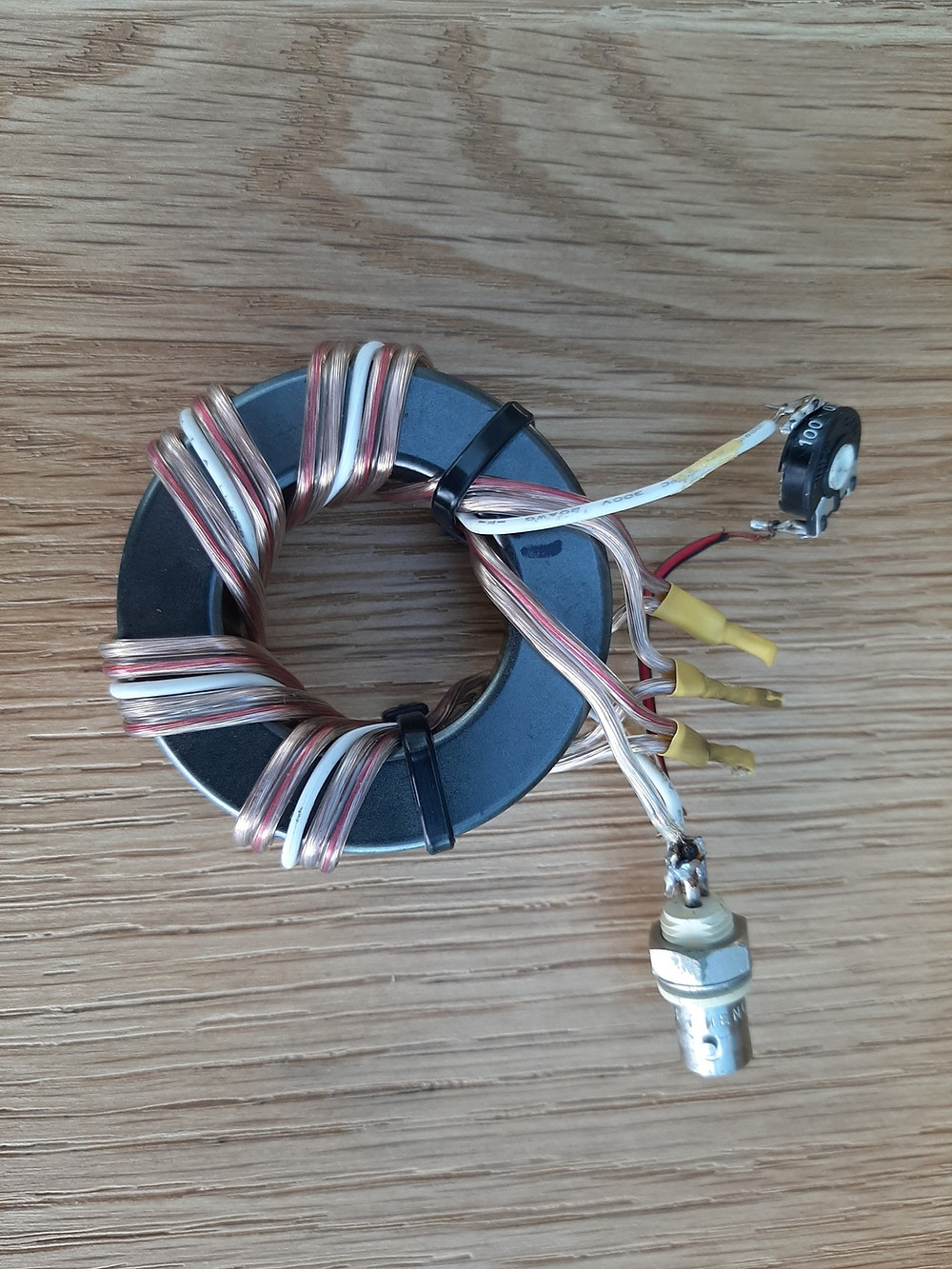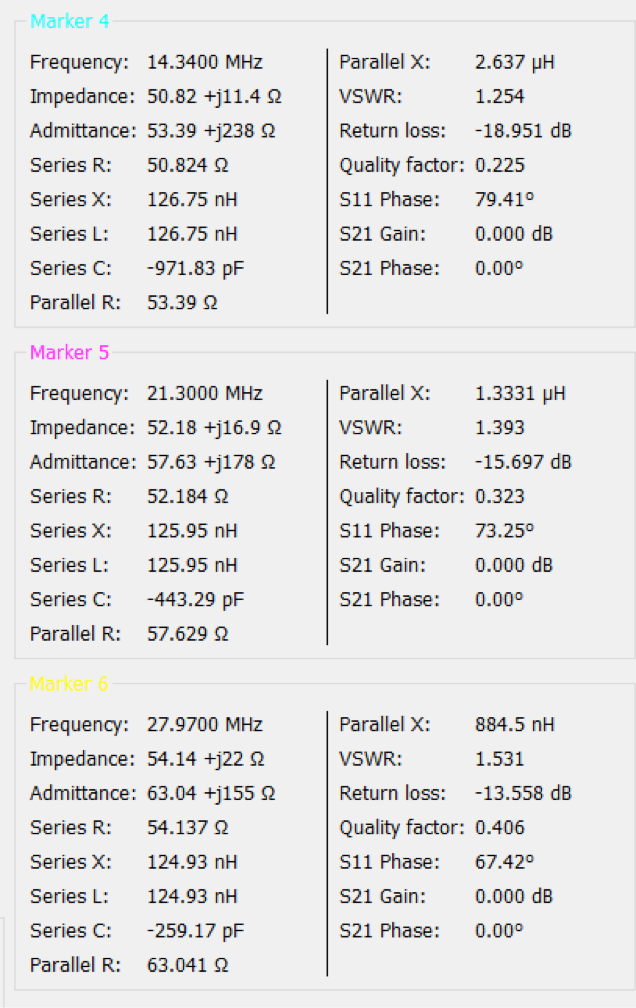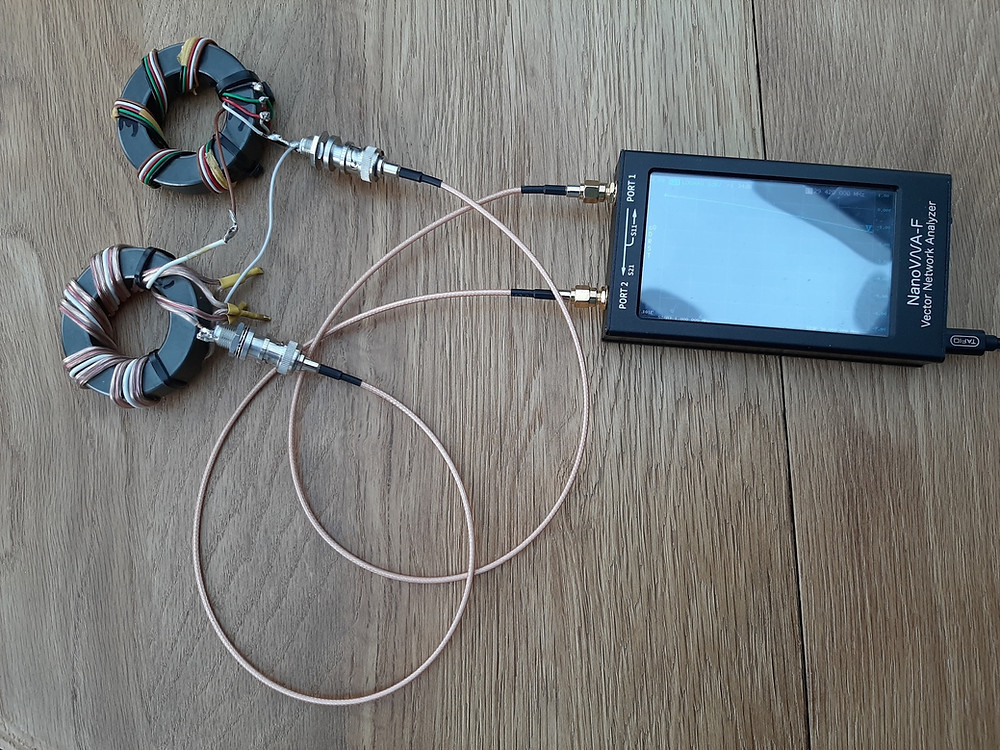Search
• xtgaby

# Building and measuring ununs

Updated: Jul 8, 2020

Remark: all return loss measurements (SWR) are with a light inductive load (adjustable resistor). Real results are always better then stated here.Used core: FT240-31 Amidon.

4 wires 6 turns each

1 wire 4 turns.

Adjustable resistor of 100 Ohm adjust for minimum SWR, disconnect the resistor and measure the value with a multi meter.

Measured value was exact 68 Ohm as calculated value.

Schematic diagram:Primary (left):

4 coils with 6 turns = 24 turns.

Secundary (right):

4 coils with 6 turns and 1 coil with 4 turns = 28 turns.

Impedance primary (50 Ohm) is 24 x 24 = 576

Impedance secundary 28 x 28 = 784

Calculated impedance secundary = (50/567) x 784 = 68 Ohm.Used core: FT240-31 Amidon.

5 wires 5 turns each.

Adjustable resistor of 100 Ohm adjust for minimum SWR, disconnect the resistor and measure the value with a multi meter.

Measured value was 76.8 Ohm calculated value is 78 Ohm.

Schematic diagram:Primary (left):

4 coils with 5 turns = 20 turns.

Secundary (right):

5 coils with 5 turns = 25 turns.

Impedance primary (50 Ohm) is 20 x 20 = 400

Impedance secundary 25 x 25 = 625

Calculated impedance secundary = (50/400) x 625 = 78 Ohm.Comparison 5 coils with 5 turns each (above) <==> 5 coils with 6 turns each (under).Used core: FT240-31 Amidon.

5 wires 6 turns each.

Adjustable resistor of 100 Ohm adjust for minimum SWR, disconnect the resistor and measure the value with a multi meter.

Measured value was 78 Ohm calculated value is 78 Ohm.

Schematic diagram:Primary (left):

4 coils with 6 turns = 24 turns.

Secundary (right):

5 coils with 6 turns = 30 turns.

Impedance primary (50 Ohm) is 24 x 24 = 576

Impedance secundary 30 x 30 = 900

Calculated impedance secundary = (50/576) x 900 = 78 Ohm.5 coils with 4 turns each.Used core: FT240-31 Amidon.

5 wires 4 turns each.

Adjustable resistor of 100 Ohm adjust for minimum SWR, disconnect the resistor and measure the value with a multi meter.

Measured value was 78 Ohm calculated value is 78 Ohm.

Schematic diagram:Primary (left):

4 coils with 4 turns = 16 turns.

Secundary (right):

5 coils with 4 turns = 20 turns.

Impedance primary (50 Ohm) is 16 x 16 = 256

Impedance secundary 20 x 20 = 400

Calculated impedance secundary = (50/256) x 400 = 78 Ohm.Insertion loss measurement.

The setup:

2 x unun, first from 50 ohm to 78 ohm, second from 78 ohm to 50 ohm.The results:I have used the device on 80 meter. Insertion loss for two devices = 0,092 dB.

Insertion loss = 0,092 / 2 = 0,046 dB.

This means a loss of 1,05 % (see conversion tables).

In practice, when you use 100 Watts, only 1 Watt is lost in our device.

From 50 to 75 ohm withh one coil.One coil 10 turns is output.

Input is 8 turns.